# Texas Go Math Grade 1 Lesson 15.3 Answer Key More Three-Dimensional Solids

Refer to our Texas Go Math Grade 1 Answer Key Pdf to score good marks in the exams. Test yourself by practicing the problems from Texas Go Math Grade 1 Lesson 15.3 Answer Key More Three-Dimensional Solids.

## Texas Go Math Grade 1 Lesson 15.3 Answer Key More Three-Dimensional Solids

Explore

Draw to sort the three-dimensional solids.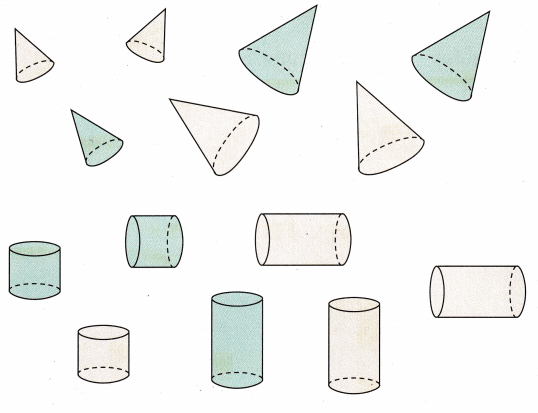FOR THE TEACHER • Have children sort the three-dimensional solids into two groups. Have them draw around each group to show how they sorted.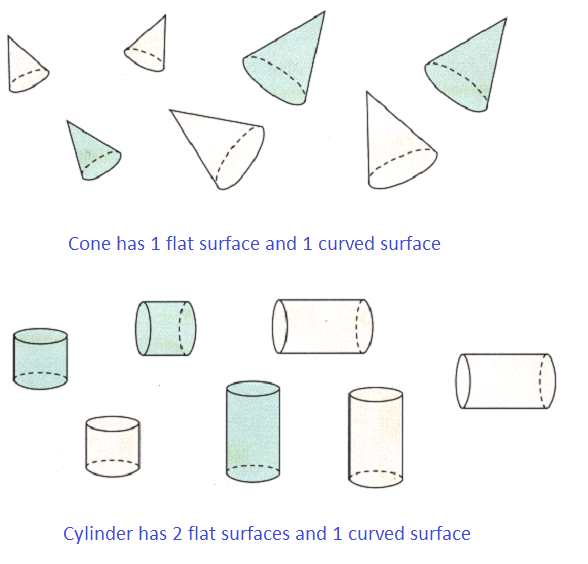Math Talk
Mathematical Processes

Explain how you sorted the solids.
Sorted the solids by differentiating the 3-dimensional objects with 1 flat surface and 2 flat surfaces.

Model and Draw

Look at the faces, the vertices, and the edges of these solids.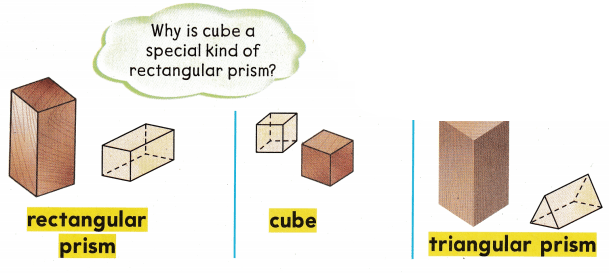THINK
A face is a flat surface of a solid.
An edge is where 2 faces meet.
A vertex is where 3 or more edges meet.

Share and Show

Use rectangular prisms, cubes, and triangular prisms. Sort the solids into groups. Name and draw the solids.

Question 1.
6 facesQuestion 2.
5 faces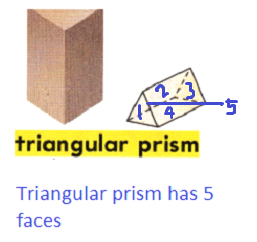Question 3.
9 edgesQuestion 4.
8 verticesProblem Solving

Use three-dimensional solids. Write how many.

Question 5.__________ faces
__________ edges
__________ vertices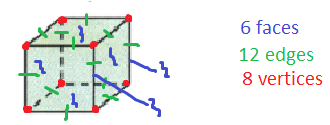Question 6.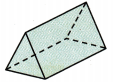__________ faces
__________ edges
__________ verticesQuestion 7.__________ faces
__________ edges
__________ verticesQuestion 8.
H.O.T. How many faces of a cube are squares?__________ faces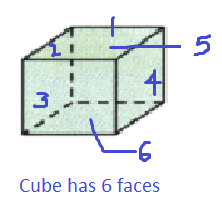Explanation:
A cube is a 3- dimensional solid object bounded by six square faces.

Question 9.
H.O.T. Multi-Step Which solid has some faces that are triangles and some faces that are rectangles? Explain.Explanation:
A triangular prism has 5 faces in which 2 are triangular faces and 3 are rectangular faces.

Question 10.
Representations Terri uses this block to build a house. What ¡s the solid?(A) cube
(B) rectangular prism
(C) cylinder
Rectangular Prism
Explanation:
A rectangular prism has rectangular faces

Question 11.
Johnny builds a birdhouse. It has 6 faces. The faces are all squares that are the same size. Which shows the shape of Johnny’s birdhouse?Question 12.
Multi-Step Louis builds an object with only triangles and rectangles. Which object does he build?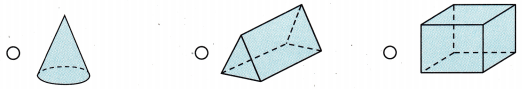Question 13.
Texas Test Prep Which solid has 5 faces?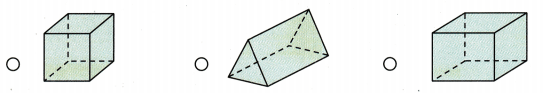Explanation:
A triangular prism has 5 faces in which 2 of them are triangular faces and 3 of them are rectangular faces.

HOME CONNECTION • Ask your child to name red objects shaped like a rectangular prism, a cube, and a triangular prism.
Rectangular prism – red colored domino,
Cube – red rubiks cube,
Triagular prism – red colored camping tent.

### Texas Go Math Grade 1 Lesson 15.3 Homework and Practice Answer Key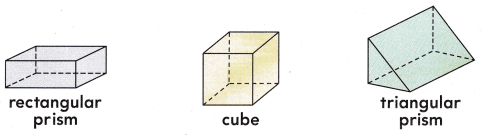Name and draw a solid to match.

Question 1.
5 facesQuestion 2.
8 vertices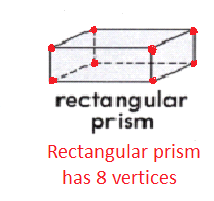Problem Solving

Question 3.
How many faces of a triangular prism are rectangles?
__________ facesExplanation:
A triangular prism has a total of 5 faces in which 2 of them are triangular faces and 3 of them are rectangular faces.

Question 4.
How many faces of a triangular prism are rectangles?
_________ facesExplanation:
A triangular prism has a total of 5 faces in which 2 of them are triangular faces and 3 of them are rectangular faces.

Question 5.
How many faces of a triangular prism are triangles?
__________ facesExplanation:
A triangular prism has a total of 5 faces in which 2 of them are triangular faces and 3 of them are rectangular faces.

Lesson Check

Question 6.
Sam finds a small cube. He counts the vertices How many does he count?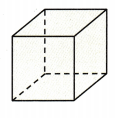(A) 5
(B) 6
(C) 8
Answer: A cube has 8 vertices, Sam counts 8 vertices in a cube.

Question 7.
Jackie chooses a triangular prism. She counts the edges. How many edges does she count?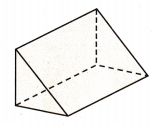(A) 9
(B) 12
(C) 6
Answer: A triangular prism has 9 edges, Jackie counts 9 edges in a triangular prism.

Question 8.
Multi-Step How many more vertices does a cube have than a triangular prism?
(A) 1
(B) 2
(C) 8
Answer: A cube has 8 vertices and a triangular prism has 6 vertices.

Question 9.
Which solid has 9 edges?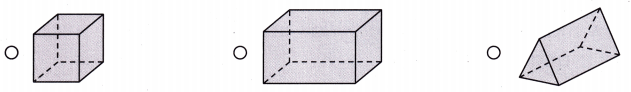Answer: A triangular prism has 9 edges.

Scroll to Top